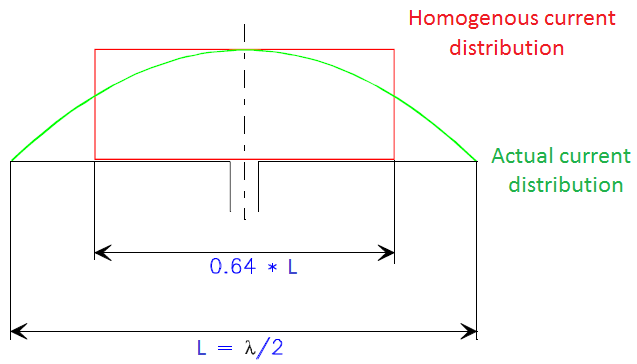# 4.2. Effective Height (Length)¶

Effective height (or length) of an air core loop in meters is [Rohner, 2006]

(4.11)$h_{eff} = \frac{ 2\pi A N }{ \lambda } = \frac{ \pi^2 D^2 N }{ 2\lambda }$

For transmitter antenna, it is a length that a dipole homogeneously carrying the feed point current I_0 would have to have in order to generate the same field strength in the main direction of radiation as

(4.12)$h_{eff} = \int_0^L \frac{ I(Z) }{ I_0 } dz$

where $$I(z)$$ is the current distribution, $$I_0$$ is the feed point current (maximum). In the case of a half-wave dipole antenna ($$I(z)=I_0 \cos⁡ {2\pi L/ \lambda}), h_{eff}=\lambda/\pi=2L/\pi=0.64L$$). This is shown Fig. 4.4.Fig. 4.4 : Explanation of the effective length concept.

For receiver antenna, the effective height of the dipole antenna is [Laurent and Carvalho, 1962]

(4.13)$h_{eff} = \frac{v_{ind}}{E} h_{eff} = \frac{\omega_0 N A_r}{C} \left[ \mu_{cer} + \biggl( \frac{d_c^2}{d_r^2} - 1 \biggr) \right]$

where $$\omega_0$$ is resonance frequency, $$N$$ is number of turns, $$A_r$$ is area of the rod, $$\mu_{cer}$$ is effective relative permeability of the rod, $$d_c$$ and $$d_r$$ are diameters of the coil and rod respectively[Laurent and Carvalho, 1962].

Therefore, for an N turn ferrite rod antenna, [Snelling, 1969]

(4.14)$h_{eff} = \mu_{cer} \frac{\omega AN}{c_0} = \mu_{cer} \frac{2\pi AN}{\lambda_0}$

Another effective height formula for ferrite loaded solenoid [Burhans, 1979]:

(4.15)$h_{eff} = \frac{2\pi AN \mu_{cer} F_a}{\lambda}$

where $$F_a$$ is averaging factor of coil and rod (typically 0.5 to 0.7).